# Introduction

Scientists often evaluate theories or draw conclusions by informally combining results from several experiments. The experiments and the measured outcomes are usually diverse – making a meta-analyses inappropriate – and scientists therefore typically use the number of significant p-values to support their conclusion. P-values, however, are a poor way of integrating results, and since statistical power is often low, “conflicting results” are common. Informal methods of evaluating a series of experiments makes inefficient use of the data and can lead to incorrect conclusions and poor decisions. Here we show how to combine diverse evidence across experiments using Bayes factors [1,2], based on a method developed by Kuiper et al. .

The procedure is outlined in Figure 1 and consists of the following five steps:

1. Before seeing the data, specify the prior probability of three hypotheses: that the effect is less than zero, greater than zero, and exactly zero. An equal probability of 1/3 is usually appropriate for these exhaustive and mutually exclusive hypotheses. These probabilities will be updated after observing the data. (It is also possible to have a range of values around zero instead of a point value of exactly zero, and the null hypothesis could be a value other than zero; we will ignore these details for now.)

2. Specify a prior distribution for the effect size (ES). The ES depends on the research question and could be a difference between means, the slope of a regression line, or an odds ratio. The BayesCombo package calculates a sensible default prior if none is specified.

3. Calculate the effect size and standard error (SE) from an experiment, which can be obtained from output of a standard statistical analysis.

4. Calculate the Bayes factor (BF) for each hypothesis, which represents the evidence for a hypothesis after seeing the data, relative to the probability of a hypothesis before seeing the data. The BFs are calculated by the BayesCombo package as a ratio of posterior to prior distributions over a defined range of parameter values.

5. Update the prior probability for each hypothesis with the Bayes factors to give the posterior probability for each hypothesis.

For the next experiment, use these updated probabilities to replace those defined in Step 1 and go through steps 2–5 again. Repeat for all experiments that you want to include. Let’s work through an example.

# Posterior probability for a single experimentFive steps to get from a prior to a posterior probability of a hypothesis. The effect size is the parameter $$\theta$$, and H<, H0, and H> represent the three hypotheses of negative, zero, and positive effects.

## Step 1: Specify priors for hypotheses

We have an experiment where 20 rats were randomised to one of four doses of the antidepressant fluoxetine, given in the drinking water. The time that the rats spent immobile in the Forced Swim Test (FST) was recorded. The FST is a standard behavioural test of “depression” in rodents.

First, we specify the prior probability of three hypotheses: that fluoxetine increases (H>), decreases (H<), or has no effect (H0) on immobility time. These three hypotheses are exhaustive (include all possible outcomes) and mutually exclusive (only one can be true). Although fluoxetine is known to decrease immobility time, we will specify an equality probability of 1/3 for each hypothesis to illustrate the approach.

## Step 2: Specify a prior for the effect size

Next, we need to specify a prior for the effect size (we define the effect size in the Step 3). For now we will use the default prior, which is calculated from the data. It is a normal prior, centred at zero, with the width calculated such that the 99% confidence interval (CI) of the prior matches the 99% CI of the data distribution. The results can be sensitive to the choice of prior, especially if the width is large relative to the data. The default prior is suitable for most situations where you have no prior information to include, and the results are insensitive to small changes near the default value (see below).

## Step 3: Calculate effect size and standard error

The data for this example are in the labstats package (available on CRAN) and plotted in Figure 2. To calculate the effect size (and the prior in the previous step) we need to define the analysis. Here, dose is treated as a continuous variable (see reference ) and so a linear regression quantifies the relationship between fluoxetine and immobility time. No effect corresponds to a flat line (slope = 0) in Figure 2.

library(labstats)
par(las=1)
plot(time.immob ~ dose, data=fluoxetine, col="royalblue",
ylab="Time immobile (s)", ylim=c(0, 250), xlim=c(0, 250),
xlab="Dose of fluoxetine (mg/L in drinking water)")
abline(lm(time.immob ~ dose, data=fluoxetine), col="royalblue") # add reg. line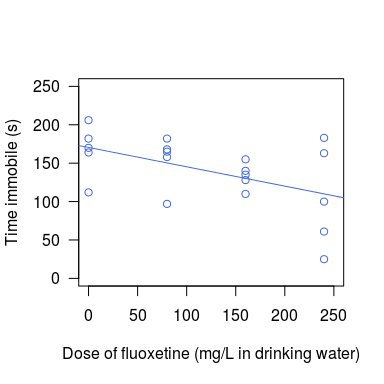Effect of fluoxetine (Prozac) on rats in the Forced Swim Test. Data are from Lazic 

The code below calculates the effect size and standard error. These are the required inputs to calculate the posterior probabilities and are returned from lm(), glm(), and related functions (e.g. from ANOVAs, t-tests, or regressions with Gaussian, Poisson, or binomial outcomes).

summary(lm(time.immob ~ dose, data=fluoxetine))coef ## Estimate Std. Error t value Pr(>|t|) ## (Intercept) 170.440 14.8368131 11.48764 1.01541e-09 ## dose -0.252 0.0991326 -2.54205 2.04365e-02 From the above output we see that the estimated slope is -0.252, with a standard error of 0.099, and a p-value of 0.020. We now have all the information to calculate the probability that fluoxetine increases, decreases, or has no effect on immobility time.1 ## Step 4: Calculate Bayes factors Bayes factors are used as an intermediate step in calculating the posterior probabilities of each hypothesis. The functions in the BayesCombo package calculate these automatically, but we take a brief digression from the fluoxetine example to illustrate how they are calculated to provide some insight into the method. Figure 3 shows a standard Bayesian updating of a prior distribution to a posterior distribution based on the data (likelihood). The prior in the top panel is normal with a mean of zero and standard deviation of 1.29. The middle panel shows the data (likelihood) distribution, which has a mean of 0.75 and standard deviation of 1. The bottom panel shows the posterior, which is shifted to positive values relative to the prior and reflects the influence of the data. The prior and likelihood correspond to steps 2 and 3 in Figure 1. The BFs for the three hypotheses are then calculated as the ratio of posterior to prior areas or heights of the distributions: $BF_{H<0} = \dfrac{\mathrm{area~of~d}}{\mathrm{area~of~a}}$ $BF_{H=0} = \dfrac{\mathrm{height~of~point~e}}{\mathrm{height~of~point~b}}$ $BF_{H>0} = \dfrac{\mathrm{area~of~f}}{\mathrm{area~of~c}}$ For example, 50% of the prior distribution is above 0 (region c), as is 72% of the posterior (region f). The interpretation is that the data have increased the plausibility of hypothesis H> from 50% to 72%. The ratio of these values is the Bayes factor and is equal to 0.72/0.5 = 1.4. Thus, we can say that H> is 1.4 times more likely. More generally, a BF > 1 means that the data support a hypothesis, whereas a BF < 1 means that data do not support a hypothesis.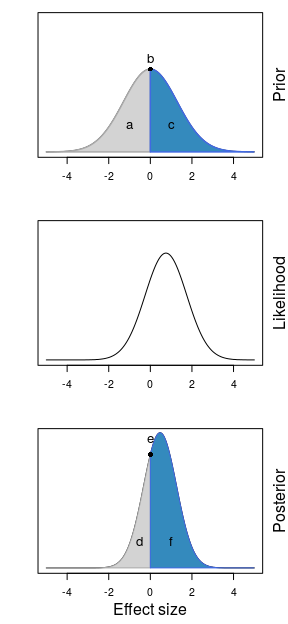Prior, Likelihood (data), and posterior distributions for an experiment. Bayes factors for the three hypotheses are calculated as the ratio of posterior to prior areas or heights. ## Step 5: Use the BFs to update the prior probability of each hypothesis The final step is to update the prior probability for each hypothesis with the BFs to get the posterior probability for each hypothesis. The equation below shows the calculation for the hypothesis that the effect is greater than zero (H>), and other probabilities are calculated in the same way, just substituting other BFs in the numerator. $$Pr()$$ are the prior probabilities for each hypothesis, and they cancel out from the equation when they are all equal (e.g. if they are all 1/3). $P(H>) = \dfrac{Pr(H>)BF_{H>}}{Pr(H<)BF_{H<} + Pr(H0)BF_{H0} + Pr(H>)BF_{H>}}$ All of the above steps can be conveniently calculated using the pph() function (Posterior Probability of a Hypothesis). Returning to the fluoxetine example, we can calculate the probability that the slope is negative, positive, or zero. Below, we specify the slope (beta = -0.252) and its standard error (se.beta = 0.099) that we obtained previously from the output of the lm() function. The default settings are used for all other options. The output below shows that the probability of a negative slope (H<) is 0.9120, a positive slope (H>) is 0.0106, and a zero slope (H0, corresponding to no effect) is 0.0774. Unlike a p-value, which is the probability of the data given a hypothesis, these probabilities have a direct and intuitive interpretation as the probability of a hypothesis given the data. x <- pph(beta = -0.252, se.beta = 0.099) summary(x) ## H< H0 H> ## 0.9120 0.0774 0.0106 Plotting the output of the pph() function returns the likelihood, prior, and posterior distributions (Fig. 4). The mean of the likelihood (data distribution; dotted line) is centred on -0.252 and the standard error (0.099) determines the width of this distribution. The prior (thick grey line) was automatically calculated and we can see how it encompasses the likelihood distribution and is centred at zero. The posterior distribution (thin black line) represents the combination of prior and likelihood. The BFs and posterior probabilities of the three hypotheses are then calculated from these prior and posterior distributions (analogous to the distributions in Fig. 3). par(las=1) plot(x, leg.loc = "topright", xlab="Effect size (slope of regression line)")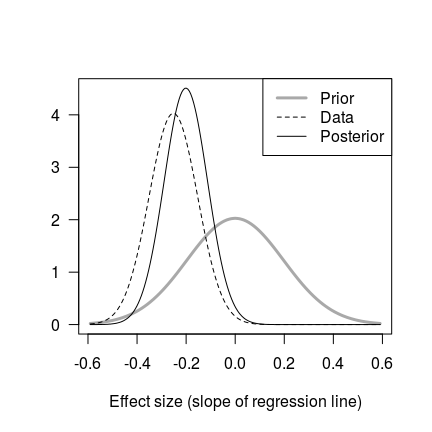Prior, likelihood (data), and posterior distributions for the fluoxetine data. The above default prior may be more informative than desired (note how the posterior is pulled towards the prior in Fig. 4) and the easiest way to decrease the influence of the prior is to make it wider by specifying a multiplier. The code below doubles the previous standard error (se.mult = 2), and the posterior is now much closer to the data distribution (Fig. 5). However, the posterior hypothesis probabilities are similar to the previous analysis; rounded to two decimal places, the probability that the slope is negative is still 0.91. x2 <- pph(beta = -0.252, se.beta = 0.099, se.mult = 2) summary(x2) ## H< H0 H> ## 0.9051 0.0887 0.0062 par(las=1) plot(x2, leg.loc = "topright", xlab="Effect size (slope of regression line)")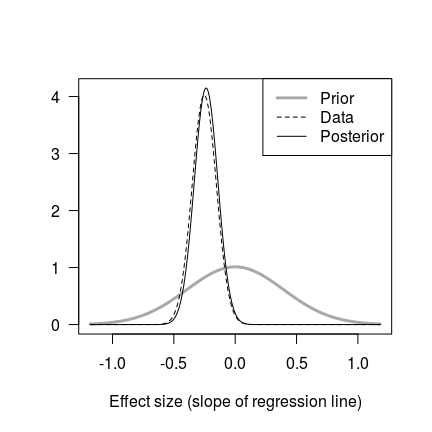Prior, likelihood (data), and posterior distributions for the fluoxetine data. Same as Fig. 4 but with a wider prior. A final example illustrates other options. The prior for the slope is directly specified as having a mean of 0 (beta0 = 0) and standard error of 1.2 (se0 = 1.2). In the previous analyses H0 was defined as exactly equal to 0, but here we define H0 as a range of values close to zero using H0 = c(-0.05, 0.05). Finally, the priors on the hypotheses are also given as an argument to H.priors. The values indicate that the prior probability of the slope being negative, zero, and positive are 0.495, 0.495, 0.01, respectively. The interpretation is that we expect that fluoxetine either decreases immobility time or has no effect, but it is unlikely to increase immobility time. x3 <- pph(beta = -0.252, se.beta = 0.099, beta0=0, se0=1.2, H0 = c(-0.05, 0.05), H.priors=c(0.495, 0.495, 0.01)) summary(x3) ## H< H0 H> ## 0.984 0.016 0.000 par(las=1) plot(x3, leg.loc = "topright", xlab="Effect size (slope of regression line)")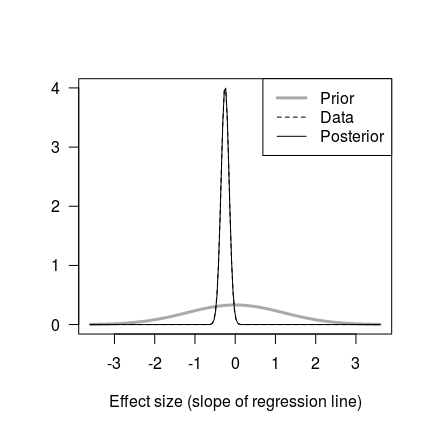Prior, likelihood (data), and posterior distributions for the fluoxetine data. Same as Fig. 4 but with alternative options specified. With these options, the probability that fluoxetine decreases immobility time is now 0.98, and the distributions are shown in Figure 6. # Posterior probability for multiple experiments We can use the above procedure to sequentially combine results from multiple experiments, where the posterior probabilities for hypotheses from one experiment are used as the prior probabilities for the next experiment. It is the analysts responsibility to ensure that the experiments are testing the same overall hypothesis or theory. In addition, the direction of the effects should be aligned; for example, if a positive effect size in one experiment is interpreted as supporting a theory, but a negative effect size in another experiment also supports the theory, then the negative effect should be multiplied by -1 to change its sign. The ev.combo() function combines results and only requires effect sizes (beta) and standard errors (se.beta) from two or more experiments as input. The default prior mean (beta0 = 0) is suitable for most analyses, as is the equal prior hypothesis probabilities (H.priors = c(1/3, 1/3, 1/3)) for each hypothesis. In the example below, assume we have four clinical trials where positive effect sizes indicate a beneficial effect of a treatment. x4 <- ev.combo(beta = c(2.3, 1.2, 0.2, 0.44), se.beta = c(1.03, 0.75, 0.16, 0.28)) The forestplot() function makes a graph resembling a traditional forest plot (Fig. 7), with the observed effect sizes and their 99% CI (black lines). The automatically calculated priors for the effect sizes are also plotted (grey lines). All four experiments have positive effect sizes but only the first experiment is significant at the usual 0.05 level, and a simple “vote counting” suggests that the treatment is ineffective, despite the three non-significant studies being in the predicted direction. par(las=1) forestplot(x4) abline(v=0, lty=2)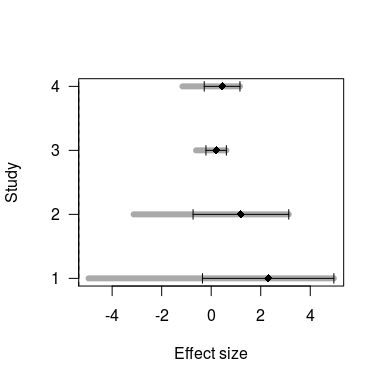Forest plot showing effect sizes for four hypothetical clinical studies. Positive effect sizes indicate an improvement and grey lines are the priors. The results summary below shows how the support for the three hypotheses changes as each experiment is added. The first line in the output contains the prior hypothesis probability of 1/3 or 33%. When the first experiment is included (second row) the probability that the effect size is greater than zero (H>) increases to 85%. As more experiments are included, the probability increases further to 98%, and the null has only 1.7% support. summary(x4) ## H< H0 H> ## [1,] 0.3333 0.3333 0.3333 ## [2,] 0.0213 0.1324 0.8464 ## [3,] 0.0022 0.0605 0.9373 ## [4,] 0.0004 0.0382 0.9614 ## [5,] 0.0000 0.0167 0.9833 It is easier to see how these probabilities change as experiments are added with a graph (Fig. 8). par(las=1) plot(x4, ylab="PPH", xlab="Study")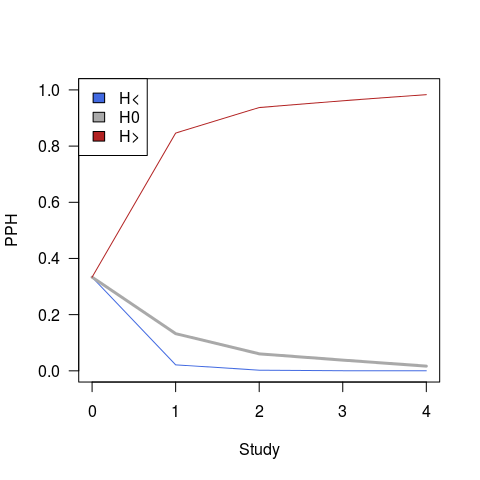Accumulation of support for the H> hypothesis as studies are added. PPH = Posterior Probability of a Hypothesis. In addition to seeing how the probabilities of hypotheses change as experiments are added, we can also plot the evidence for each experiment. This is equivalent to analysing each experiment separately with the pph() function, and the results are shown in Fig. 9. Both graphs plot the same data; the left graph group the studies by hypothesis, while the right graph groups the hypotheses by study. It is easy to see in the left graph that the H> hypothesis has the most support (above 0.5) for all four experiments. par(mfrow=c(1,2)) dotchart(x4pph.uniform, xlim=c(0,1), xlab="PPH", pch=21, bg="grey")
dotchart(t(x4\$pph.uniform), xlim=c(0,1), xlab="PPH", pch=21, bg="grey")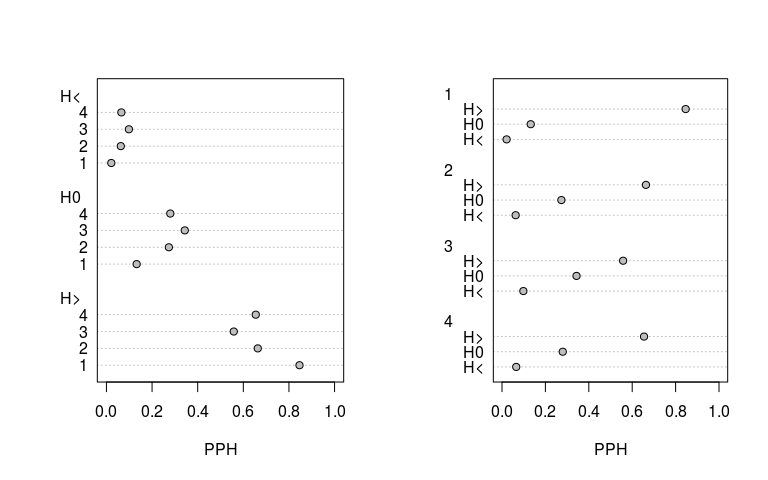Posterior Probabilities for each hypothesis for four studies. Each graph shows the same information and only groups the hypothesis and studies differently.

1. A potential source of confusion: We use normal or Gaussian distributions to represent likelihoods and priors, and normal distributions are defined by a mean and standard deviation. A standard error reflects the uncertainty (or precision) of an estimate, which we get from the output of a standard statistical analysis. Thus, we can say that “the estimated slope is -0.252 with a standard error of 0.099”. We can also say that we will represent our uncertainty in the slope as a “normal distribution with a mean of -0.252 a standard deviation of 0.099”. Here we are saying that a standard error and standard deviation are the same value, which is not usually true. We can do this because we are using the output of one analysis as the input into another one. Later in the code you will see the likelihood defined as beta = -0.252 and se.beta = 0.099. Similarly, the prior for the effect size is defined as beta0 and se0. But both se.beta and se0 are the standard deviations of normal distributions (e.g. as in Fig. 4). This confusion in terminology arises because we are taking the output from a frequentist analysis and using it as input into a Bayesian analysis. To keep things simple, we have used se in the code when referring to this quantity.